# Norms of Ideals

In this section we extend the notion of norm to ideals. This will be helpful in proving of class groups in the next section. For example, we will prove that the group of fractional ideals modulo principal fractional ideals of a number field is finite by showing that every ideal is equivalent to an ideal with norm at most some a priori bound.

Definition 10.3.1 (Lattice Index)   If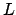and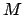are two lattices in vector space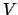, then the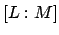is by definition the absolute value of the determinant of any linear automorphism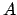ofsuch that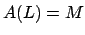.

The lattice index has the following properties:

• If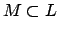, then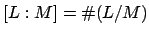.
• If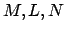are lattices then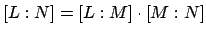.

Definition 10.3.2 (Norm of Fractional Ideal)   Suppose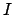is a fractional ideal of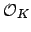. The ofis the lattice index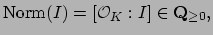or 0 if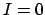.

Note that ifis an integral ideal, then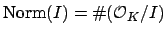.

Lemma 10.3.3   Suppose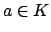andis an integral ideal. Then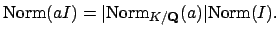Proof. By properties of the lattice index mentioned above we have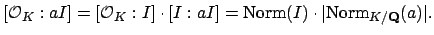Here we have used that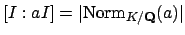, which is because left multiplication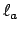is an automorphism of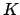that sendsonto, so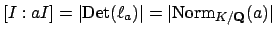.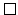Proposition 10.3.4   Ifand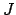are fractional ideals, then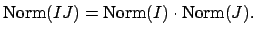Proof. By Lemma 10.3.3, it suffices to prove this whenandare integral ideals. Ifandare coprime, then Theorem 9.1.3 (Chinese Remainder Theorem) implies that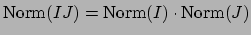. Thus we reduce to the case when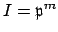and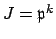for some prime ideal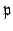and integers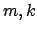. By Proposition 9.1.8 (consequence of CRT that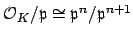), the filtration of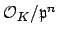given by powers ofhas successive quotients isomorphic to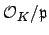, so we see that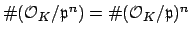, which proves that.Lemma 10.3.5   Fix a number field. Let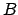be a positive integer. There are only finitely many integral idealsofwith norm at most.

Proof. An integral idealis a subgroup ofof index equal to the norm of. If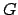is any finitely generated abelian group, then there are only finitely many subgroups ofof index at most, since the subgroups of index dividing an integer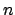are all subgroups ofthat contain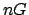, and the group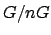is finite. This proves the lemma.William Stein 2004-05-06